# Java Assignment Related Message digest n Message Digest

• Slides: 11Java Assignment Related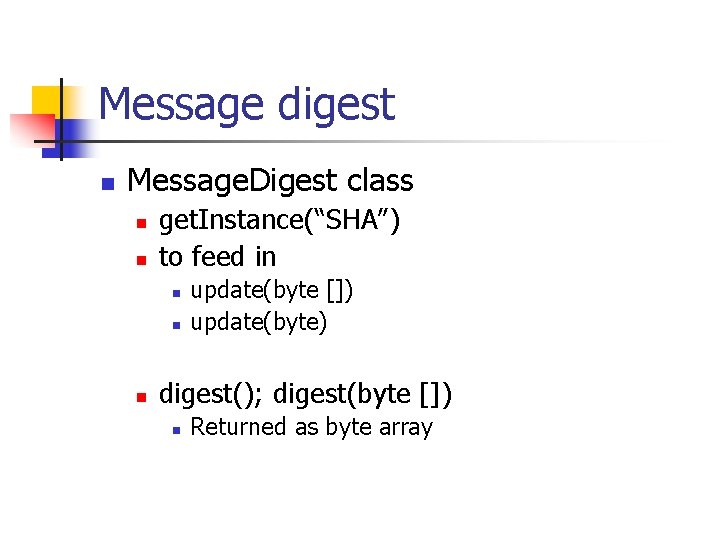Message digest n Message. Digest class n n get. Instance(“SHA”) to feed in n update(byte []) update(byte) digest(); digest(byte []) n Returned as byte array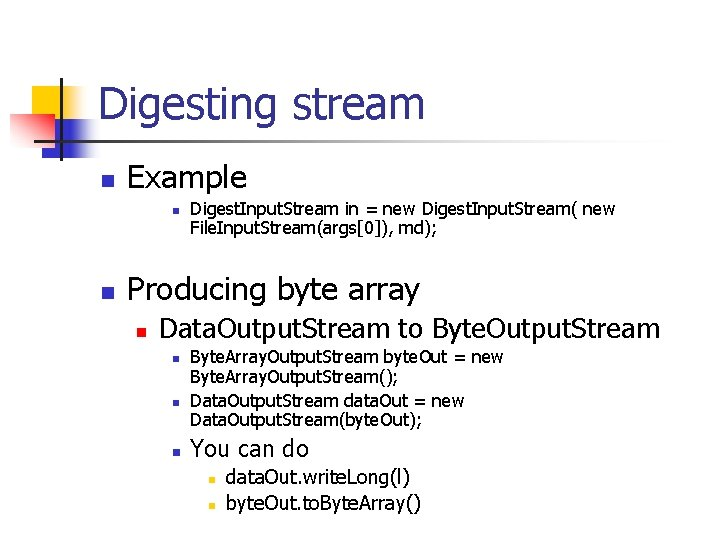Digesting stream n Example n n Digest. Input. Stream in = new Digest. Input. Stream( new File. Input. Stream(args), md); Producing byte array n Data. Output. Stream to Byte. Output. Stream n n n Byte. Array. Output. Stream byte. Out = new Byte. Array. Output. Stream(); Data. Output. Stream data. Out = new Data. Output. Stream(byte. Out); You can do n n data. Out. write. Long(l) byte. Out. to. Byte. Array()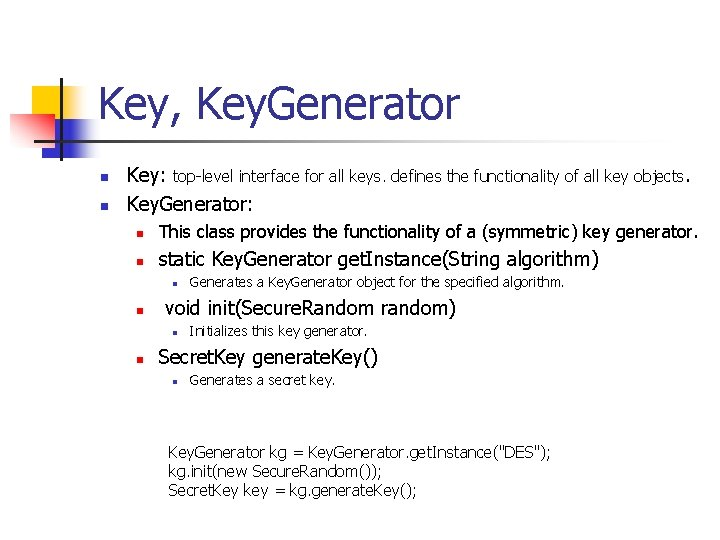Key, Key. Generator n n Key: top-level interface for all keys. defines the functionality of all key objects. Key. Generator: n This class provides the functionality of a (symmetric) key generator. n static Key. Generator get. Instance(String algorithm) n n void init(Secure. Random random) n n Generates a Key. Generator object for the specified algorithm. Initializes this key generator. Secret. Key generate. Key() n Generates a secret key. Key. Generator kg = Key. Generator. get. Instance("DES"); kg. init(new Secure. Random()); Secret. Key key = kg. generate. Key();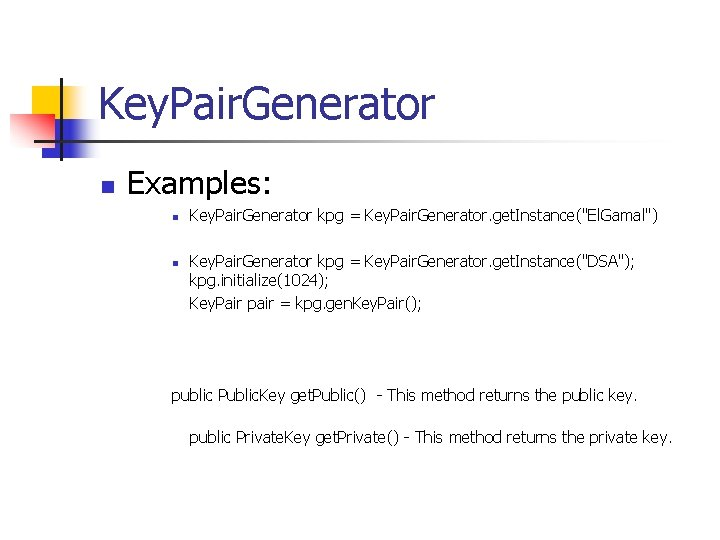Key. Pair. Generator n Examples: n n Key. Pair. Generator kpg = Key. Pair. Generator. get. Instance("El. Gamal") Key. Pair. Generator kpg = Key. Pair. Generator. get. Instance("DSA"); kpg. initialize(1024); Key. Pair pair = kpg. gen. Key. Pair(); public Public. Key get. Public() - This method returns the public key. public Private. Key get. Private() - This method returns the private key.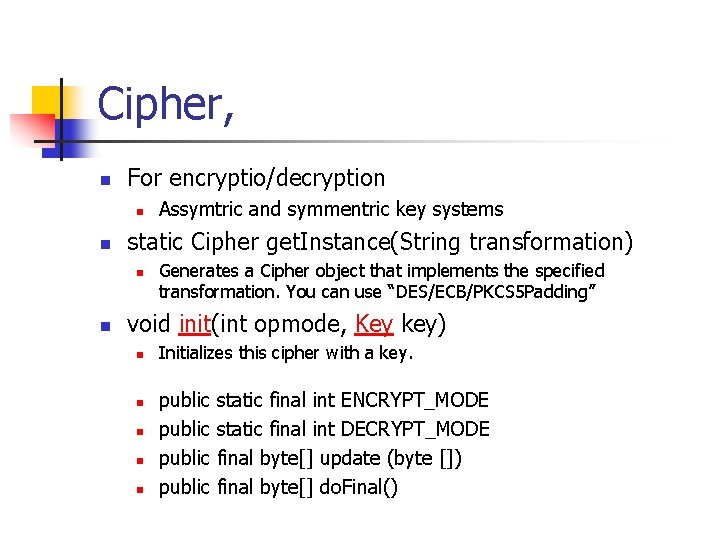Cipher, n For encryptio/decryption n n static Cipher get. Instance(String transformation) n n Assymtric and symmentric key systems Generates a Cipher object that implements the specified transformation. You can use “DES/ECB/PKCS 5 Padding” void init(int opmode, Key key) n n n Initializes this cipher with a key. public static final int ENCRYPT_MODE public static final int DECRYPT_MODE public final byte[] update (byte []) public final byte[] do. Final()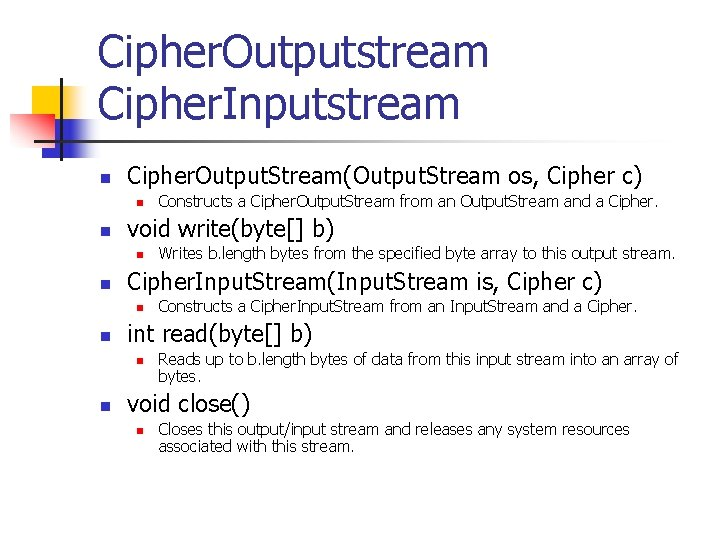Cipher. Outputstream Cipher. Inputstream n Cipher. Output. Stream(Output. Stream os, Cipher c) n n void write(byte[] b) n n Constructs a Cipher. Input. Stream from an Input. Stream and a Cipher. int read(byte[] b) n n Writes b. length bytes from the specified byte array to this output stream. Cipher. Input. Stream(Input. Stream is, Cipher c) n n Constructs a Cipher. Output. Stream from an Output. Stream and a Cipher. Reads up to b. length bytes of data from this input stream into an array of bytes. void close() n Closes this output/input stream and releases any system resources associated with this stream.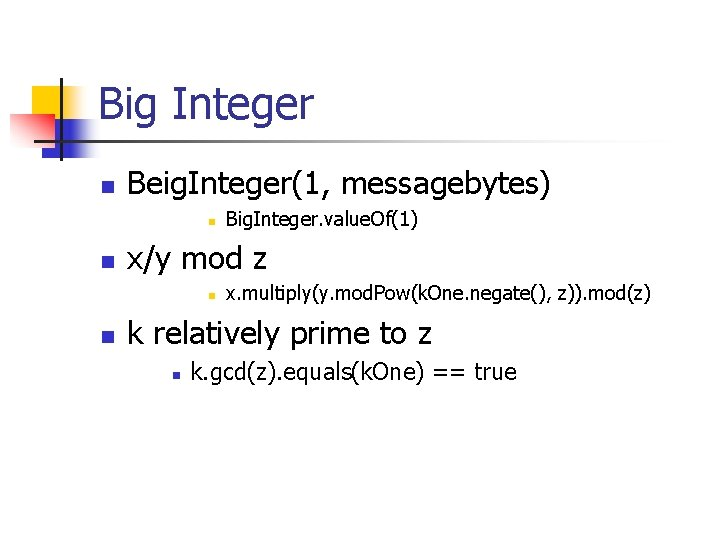Big Integer n Beig. Integer(1, messagebytes) n n x/y mod z n n Big. Integer. value. Of(1) x. multiply(y. mod. Pow(k. One. negate(), z)). mod(z) k relatively prime to z n k. gcd(z). equals(k. One) == true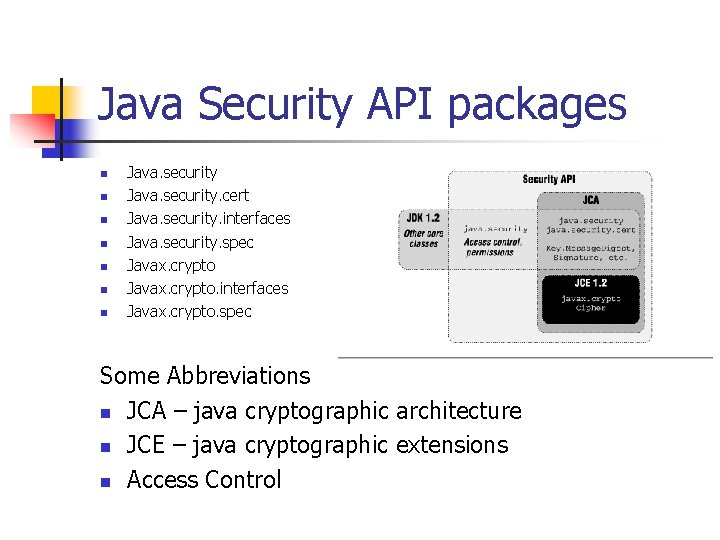Java Security API packages n n n n Java. security. cert Java. security. interfaces Java. security. spec Javax. crypto. interfaces Javax. crypto. spec Some Abbreviations n JCA – java cryptographic architecture n JCE – java cryptographic extensions n Access ControlClass or Interface Description java. security. cert. Certificate A cryptographic certificate javax. crypto. Cipher A cipher java. security. Key , java. security. Private. Key , java. security. Public. Key , javax. crypto. Secret. Key A key, used for signing or encryption javax. crypto. Key. Agreement A secret key exchange protocol java. security. Key. Factory Translates public and private keys from one format to another javax. crypto. Key. Generator Creates keys for symmetric ciphers java. security. Key. Pair. Generator Creates pairs of public and private keys for signing or encryption javax. crypto. Mac A Message Authentication Code (MAC) java. security. Message. Digest A cryptographic hash function javax. crypto. Secret. Key. Factory Translates secret keys from one format to another java. security. Secure. Random A cryptographically strong random number engine java. security. Signature A digital signature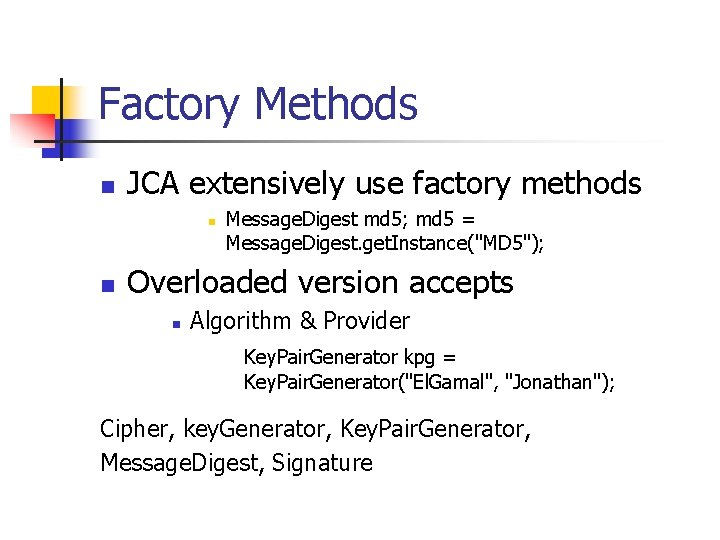Factory Methods n JCA extensively use factory methods n n Message. Digest md 5; md 5 = Message. Digest. get. Instance("MD 5"); Overloaded version accepts n Algorithm & Provider Key. Pair. Generator kpg = Key. Pair. Generator("El. Gamal", "Jonathan"); Cipher, key. Generator, Key. Pair. Generator, Message. Digest, Signature